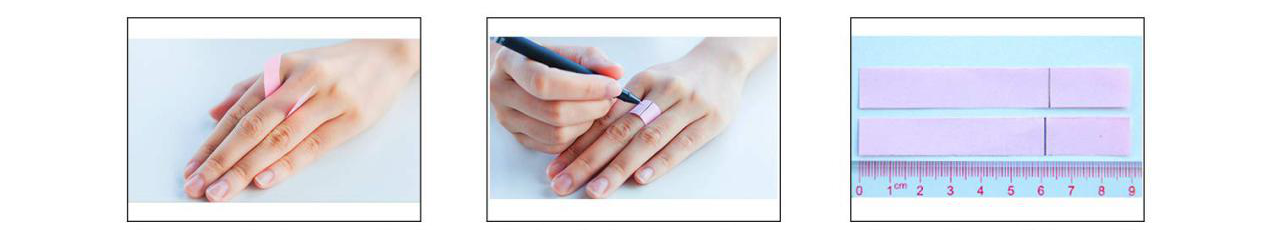Ring Size Guide

Worried about how you’ll get the measurements? Don’t panic just yet. There is a method you can use to know how to measure ring size.1. METHOD: USE A STRIP OF PAPER

To measure the ring size using a strip of paper, you will need the following:

• A strip of paper
• Ruler
• Pen/marker

2. How to measure:

• Get a non-Stretchy string or paper about 6” (150mm) long and 1/4”(6.5mm) wide
• Fold the thin strip of paper around the finger at the base and close enough to your knuckle.
• Mark the area where the two ends of the paper strip meet.
• Measure the length of the string/paper from the starting point to the mark.
• Divide the circumference (length) of the string by pi to get the incide diameter. (For example, if you know the circumference of the string is 56mm, work out 56 ÷ 3.14 = 17.8. The diameter is 17.8mm.
• Use the ring size chart above to translate the measurements into a ring size.

1. METHOD: USE A STRIP OF PAPER

To measure the ring size using a strip of paper, you will need the following:

• A strip of paper
• Ruler
• Pen/marker

2. How to measure:

• Get a non-Stretchy string or paper about 6” (150mm) long and 1/4”(6.5mm) wide
• Fold the thin strip of paper around the finger at the base and close enough to your knuckle.
• Mark the area where the two ends of the paper strip meet.
• Measure the length of the string/paper from the starting point to the mark.
• Divide the circumference (length) of the string by pi to get the incide diameter. (For example, if you know the circumference of the string is 56mm, work out 56 ÷ 3.14 = 17.8. The diameter is 17.8mm.
• Use the ring size chart above to translate the measurements into a ring size.

Ring Sizes in Different Countries

If you have any questions about your ring size, send us an email official@ciunofor.com and we will get back to you as soon as possible.